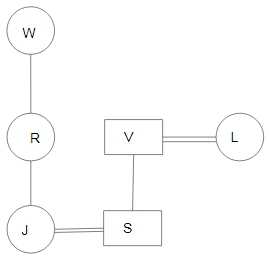Question 25

# $$A + B$$  means ‘A is the husband of B’;$$A - B$$ means ‘A is the wife of B’;$$A \times B$$ means ‘A is the mother of B’:$$A \div B$$ means ‘A is the son of B’.If, $$W \times R \times J- S \div V + L$$. then which of the following statements is NOT correct?

Solution

In the diagram, Circle shows the female, square shows the male, vertical lines show the generation, single horizontal line shows the brother/sister and double horizontal lines show the couple.From the diagram,
Option D is not correct.
$$\therefore$$ The correct answer is option D.